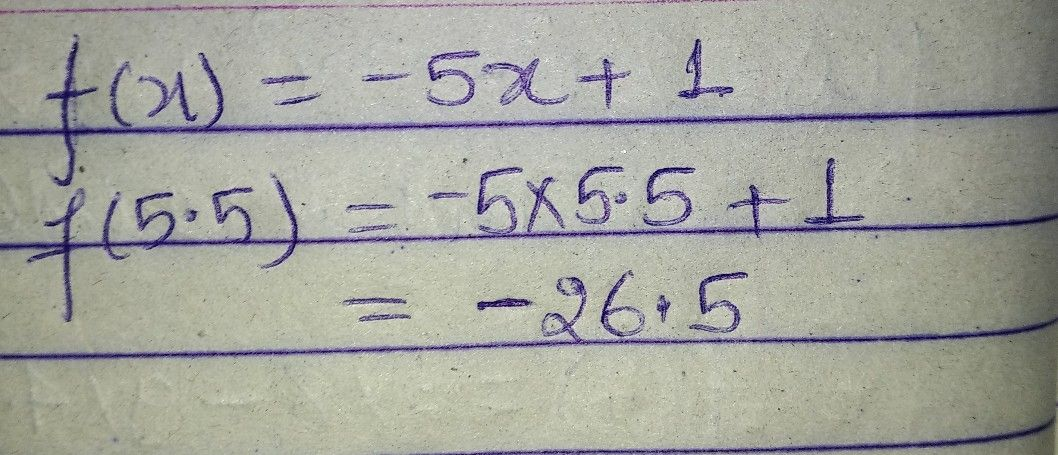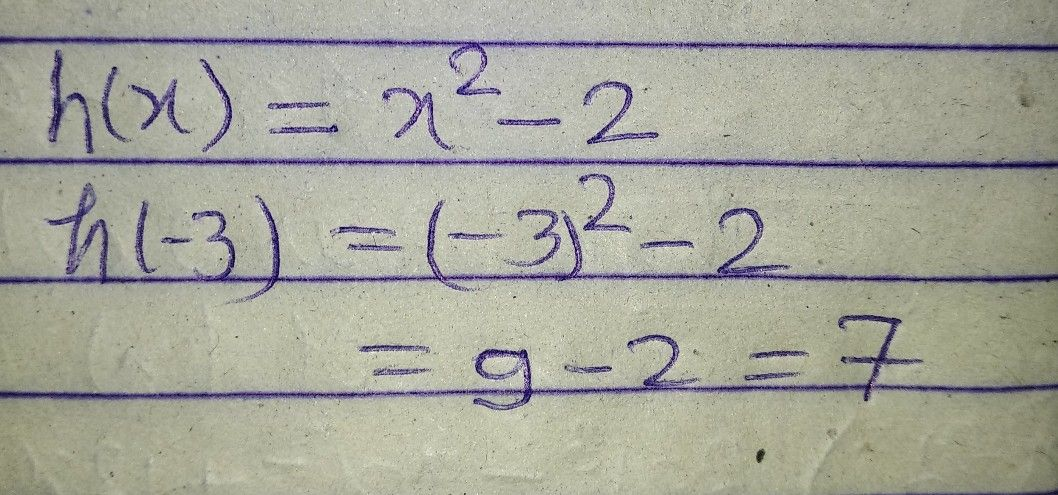Symbol
Problem$1$ 1 point $3$ Given $1f\left(x\right)=$ $-5x+1,$ what is the value of $f\left(5.5\right)7$ type your answer.. Previous
7th-9th grade
Algebra
Search count: 110
SolutionQanda teacher - ANANDStudent
Given h (x) x^2 -2 what is the value of h (-3)?Qanda teacher - ANANDdo event debate and give me extra coin for solving 2 question
and if you want to ask more question then ask me separately as preferred match question